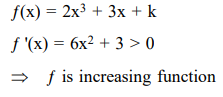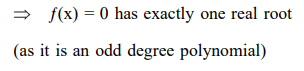# Solve this following`
Question:

The real number $\mathrm{k}$ for which the equation $2 \mathrm{x}^{3}+3 \mathrm{x}+\mathrm{k}=0$ has two distinct real roots in $[0,1]$

1. lies between 1 and 2 .

2. lies between 2 and 3 .

3. lies between $-1$ and 0

4. does not exist

Correct Option: , 4

Solution: